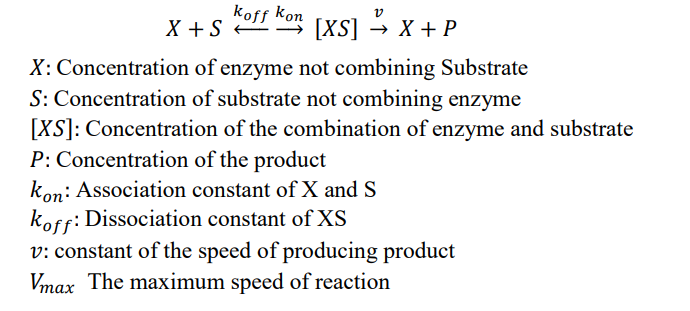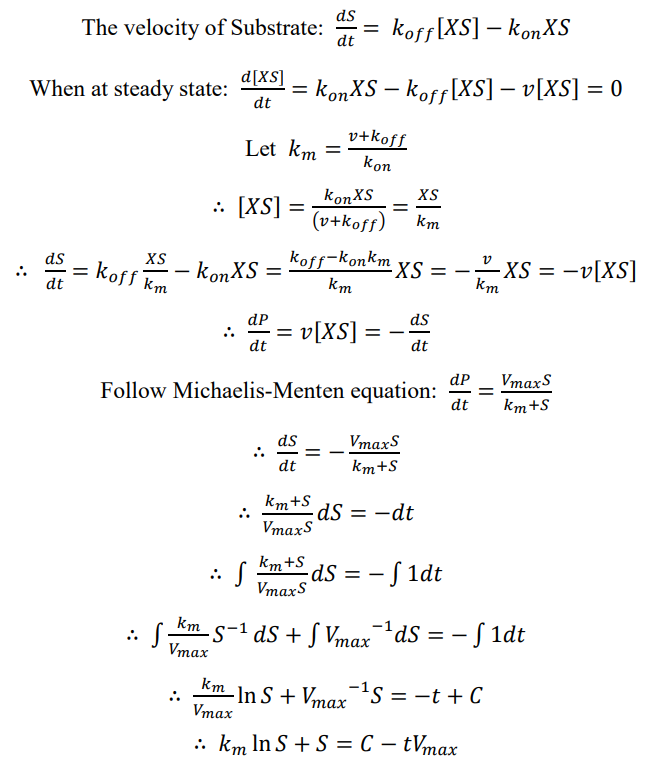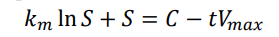# Team:SHSBNU China/Model

Model# Model

## I. Model

### a. Introduction

This model was created for describing the relationship between the concentration of the textile dye and the time.

The reaction between enzyme and the substrate can be described by the following diagram:### b. Mathematical Proof### c. Conclusion

Therefore, the relationship between concentration of textile dye and time can be described by the equation:As shown in the equation, the concentration of textile dye decreases in a natural logarithm relationship when time increases.

In the future developing, experiments need to be done to collect data and make regression for this function. Also, the function can be further developed by using Hill equation and Michaelis-Menten equation to estimate at the situation in which multiple enzymes on one PHA particle react with the substrate.